Next: Source Code Up: An Example: Computing Previous: Introduction   Contents

## Example: Computing the Matrix of Frobenius for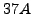at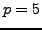Letand consider the elliptic curve, with minimal model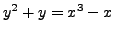.

Step 1.
Put the elliptic curve into Weierstrass form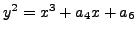, via the transformation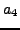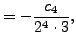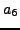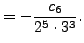In our case, we obtain the curve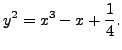Let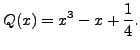Step 2.
Fix the precision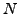and compute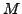. In our case,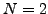and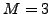.
Step 3.
Compute the action of Frobenius on the two differentials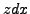and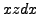as an element of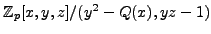, with a precision ofdigits. Furthermore, group the terms of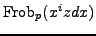as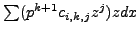, where the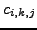are in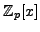of degree less than 3.

In our case, we compute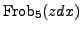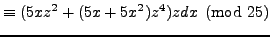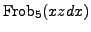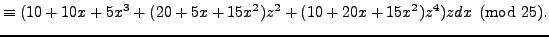Step 4.
Now we must reduce the differentials. We want to write each of the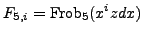as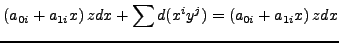in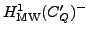. We begin with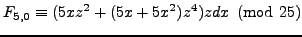and compute the appropriate list of differentials: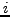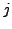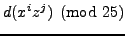0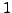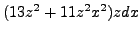1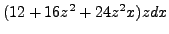2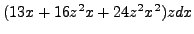0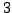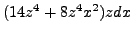1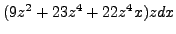2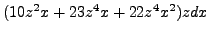Thus we wish to write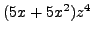as a linear combination of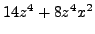,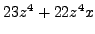, and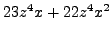, all modulo 25 (we may ignore the lower powers of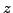present in the differentials, as we will take care of them in the steps to come). We find that taking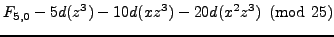leaves us with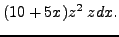Now we wish to write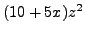as a linear combination of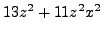,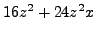, and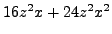, modulo 25. We find that taking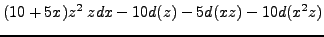leaves us with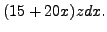Next, we reduce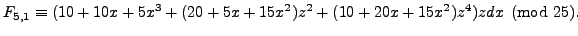Note that this has an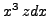term, so we take care of this first: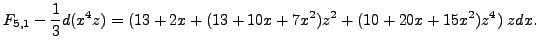Now we proceed as in the case of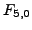, and we wish to write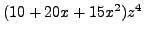as a linear combination of,, and, all modulo 25. We find that taking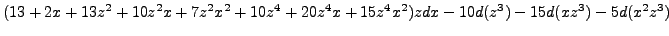leaves us with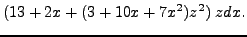Finally, we wish to write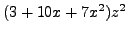as a linear combination of,, and, all modulo 25. We find that taking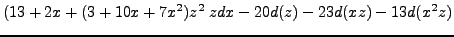leaves us with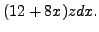Step 5.
Now we form the matrix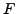of the reduced differentials, where each reduced differential gives us a column in the matrix of absolute Frobenius. In our case, we have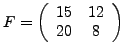.
As a consistency check, we have thathas trace 23, which is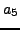modulo 25 and determinant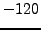, which ismodulo 25.Next: Source Code Up: An Example: Computing Previous: Introduction   Contents
William Stein 2006-10-20